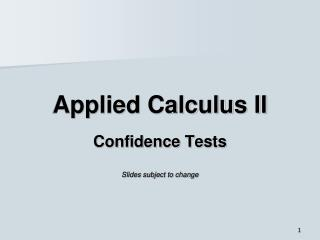# Applied Calculus II - PowerPoint PPT PresentationDownload PresentationApplied Calculus II

Applied Calculus IIDownload Presentation## Applied Calculus II

- - - - - - - - - - - - - - - - - - - - - - - - - - - E N D - - - - - - - - - - - - - - - - - - - - - - - - - - -
##### Presentation Transcript

1. Applied Calculus II Confidence Tests Slides subject to change

2. Central Limit Theorem • Draw a simple random sample of size N from any population with mean μ and standard deviation σ. • When N is large, the distribution of the sample meanXmean is approximately normal. • Or, in other words, Xmean distribution is approximately that of a normal distribution, withσmean = σ/sqrt N.

3. 1 2 3 4 5 Example 1 Normal Population • Random samples of a Normal population, N = 10. • Population characteristics are μ= 5, σ= 2. Mean

4. Example 1 Normal Population • Sample size N = 10. The central limit theorem says that the means of each sample of size N will have a normal distribution with standard deviation of σ/sqrt N. • σ is the standard deviation of the population. • The standard deviation of the means is σmean = σ/sqrt N = 2/sqrt 10 = 0.63 • In the five samples we took, the means were 5.2, 4.9, 4.9, 4.0, 5.3. • Expect the “mean of the means” to be μ (= 5.0).

5. Example 1 Normal Population • σmean = 2/sqrt 10 = 0.63 • Expect approximately 68% of the mean measurements within • Xmean– σmean and Xmean + σmean • Or, between4.37 and 5.63.

6. Samples of Normal Population 6.0 +σmean μ 5.0 −σmean 4.0 1 2 3 4 5 Mean of each sample

7. Statistical Inference • Statistical inference provides methods for drawing conclusions about a population from sample data. • One method: Normal Deviate (or z−) Test.

8. Example: Normal Deviate Test • A population of critters has mean body mass μ= 125 g and standard deviation σ = 30 g. • You hypothesize that a diet treatment increases body mass. • You apply the treatment to 10 critters and find mean body mass of the 10 critters = 142 g. • Where does this mean lies within the normal curve of our reference population? • Confidence of 95% if the mean lies above the top 5% of all sample means.

9. Normal Deviate or z−Test • Standard deviation of the sample means is σx= 30/sqrt 10 = 9.49 • z = (Xavg − μ)/ σx = (142 − 125)/ 9.49 = 1.79 • Use Table A, “C” column. Area = 0.0367. • The mean of this sample exceeds our confidence level − is in the 96.33 percentile. The diet treatment does increase body mass.

10. Example • A population of students score µ = 75 points on a standard exam with σ = 20 points. • You hypothesize that taking the exam early in the day affects performance on the exam. • You apply the treatment to 15 students and find mean score of sample = 66 points. • We have 95% confidence in our hypothesis if the sample mean is below 5th percentile or above the 95th percentile.

11. Normal Deviate or z−Test • Standard deviation of sample means is σx= 20/sqrt 15 = 5.16 • z = (Xavg − μ)/ σx = (66 − 75)/ 5.16 = −1.74 • Table A area = 0.0409 • This is only the percentile. The mean of this sample exceeds our confidence level − is in the 4.09 percentile. Taking the exam early in the day does affect performance on the exam

12. Exercise • Analyze the last 4 digits in each social security number. Find the average of each sample, N = 4. • The population is 0, 1, 2, 3, 4, 5, 6, 7, 8, 9. • For this population μ = 4.50, σ = 2.87. • The mean of the sample means should be close to 4.50. True? • The standard deviation of the sample means is σmean = σ/sqrt 4 = 1.43. • Approximately 68% of the sample means 3.07 ≤ xmean ≤ 5.93. True? If not, would finding more social security numbers help?